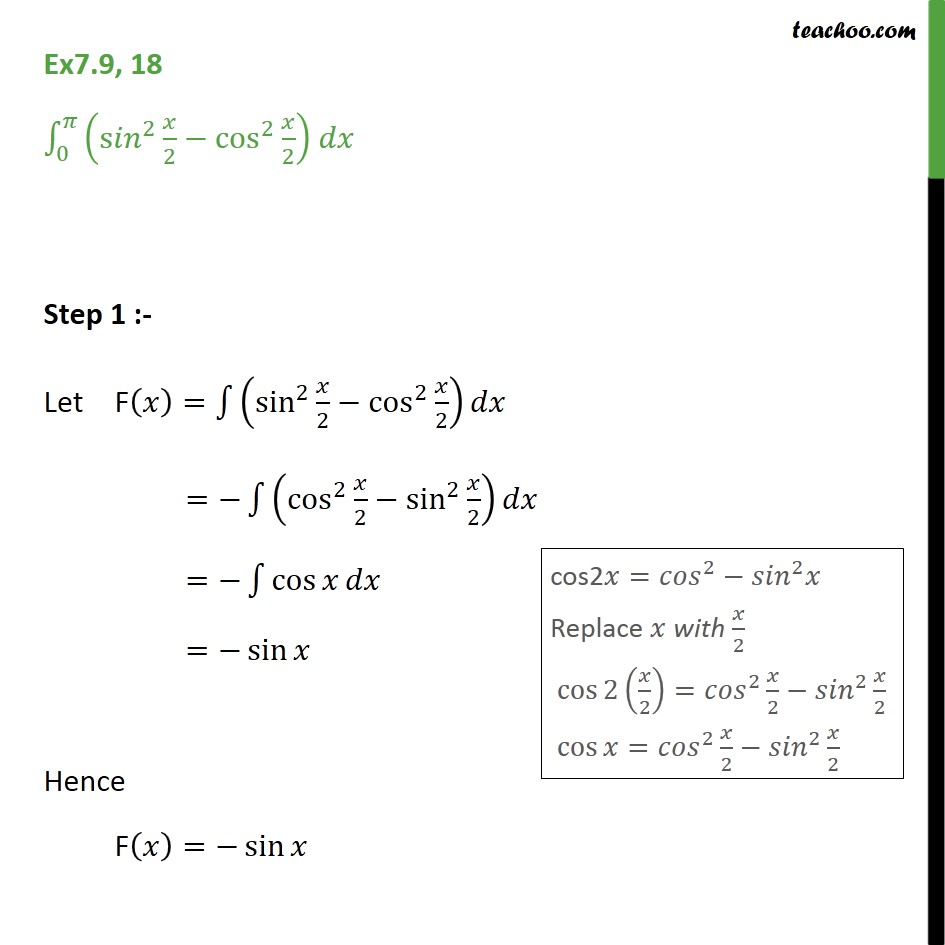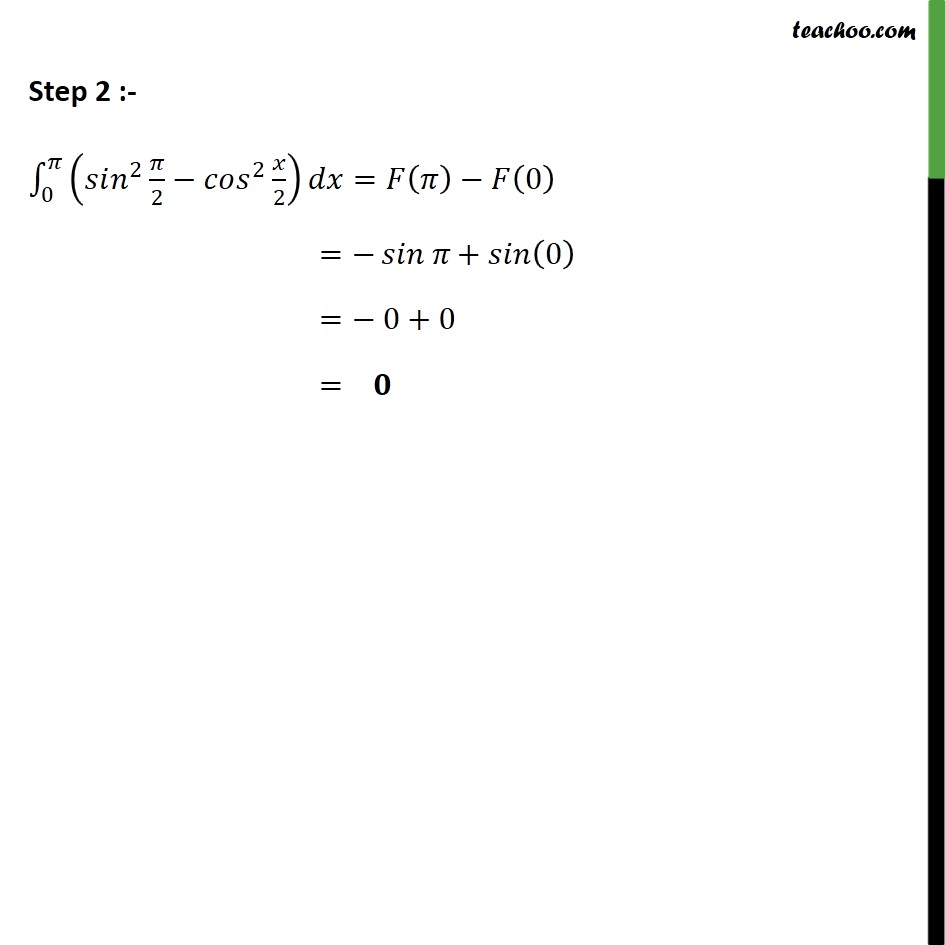Integration Full Chapter Explained - https://you.tube/Integration-Class-121. Chapter 7 Class 12 Integrals
2. Concept wise
3. Definite Integration - By Formulae

Transcript

Ex7.9, 18 0 s 2 2 cos 2 2 Step 1 :- Let F = sin 2 2 cos 2 2 = cos 2 2 sin 2 2 = cos = sin Hence F = sin Step 2 :- 0 2 2 2 2 = 0 = + 0 = 0+0 =

Definite Integration - By Formulae